# Frequency Domain Representation or Spectrum of AM Wave

## Frequency-Domain Representation or Spectrum of AM Wave

Frequency-domain representation means description of a signal with respect to its frequency  and a spectrum analyzer is a frequency-domain instrument.

### Mathematical Expressions and Wave forms

If x(t) is modulating signal and carrier signal is given by the expression :

c (t) = A cos ωt …………(1)

Then the equation for AM wave will be

s(t) = x(t) cos ωt + A cos ωt …………(2)

This equation describes the AM wave in time-domain.

However, if we want to know the frequency description or frequencies present in AM wave, we will have to find its spectrum or frequency domain representation.

For this purpose, first we have to take the Fourier transform of AM wave.

Let S(ω) denote the Fourier transform of s(t), C(ω) denotes the Fourier transform of c(t) and X(ω) denotes the Fourier transform of x(t).

Let the modulating signal x(t) and its Fourier transform X(ω) be as shown in figure 1(a).Fig. 1

Let the modulating signal or message signal x(t) be band-limited to the interval –ωm ≤ ω ≤ ωm as shown in figure.1(a).This means that the modulating signal does not have any frequency component outside the interval (–ωmm).

It may be noted at this point that in figure. 1(a) the modulating signal frequency ranges extend from – ωm to ωm i.e., it includes negative frequencies also from –ωm to 0. Practically there is no meaning of negative frequency. In fact, the negative frequency is used for mathematical convenience only.

Hence we can say that the modulating signal contains frequencies from 0 to ωm or simply the bandwidth of modulating signal is ωm.

We know that the Fourier transform of a cosine signal cos ωc t consists of two impulse at ωc and –ωc as

Since the carrier signal is c(t) = A cos ωc t, therefore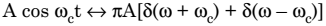……………….(4)

Figure 1 (b) shows the carrier signal A cos ωct and its Fourier transform.

Now, the AM wave is given as

s(t) = x(t) cos ωc t + A cos ωc t …………….(5)

To find the spectrum of AM wave, we take its Fourier transform.

The Fourier transform of s(t) may be found by considering the two factors x(t) cos ωc t and A cos ωc t separately as follow:

To find the Fourier transform of x(t) cos ωct, we first note the frequency-shifting theorem of Fourier transform as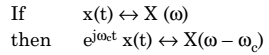………………………….(6)

This property states that if a signal x(t) is multiplied by ejωct in time-domain, then its spectrum X(ω) in frequency-domain is shifted by an amount ωc.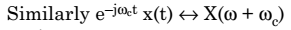……………………… (7)

But, since ejωct  is not a real function and can not be generated practically, therefore frequency shifting in practice is achieved by multiplying x(t) by a sinusoid such as cos ωc t. Therefore,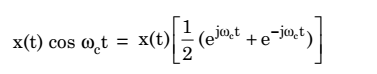………………….(8)

Hence, using equations (6) and (7), we get……………………(10)

This means that the multiplication of a signal x(t) by a sinusoid of frequency ωc shifts the spectrum X(ω) by ± ωc.

The Fourier transform of the second factor A cos ωct will be as in equation (4)……………………(11)

This means that Fourier transform of A cos ωct consists of two impulses at ± ωc.

Therefore, the Fourier transform of AM wave given by equation (2) will be the sum of equations (10) and (11). Thus,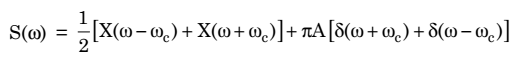………………………….(12)

Above equation for Amplitude Modulated Wave contains two factors. The first factor given as 1/2 [X(ω – ωc) + X(ω + ωc)] represents the spectrum of original or baseband signal shifted in the positive as well as in the negative direction by the factor ωc.

On the other hand, the second factor given as πA[δ(ω + ωc) + δ(ω – ωc)] represents the presence of carrier signal i.e., two impulses each having strength equal to πA. Thus the spectrum of modulated signal contains shifted spectrum of modulating signal and the spectrum of carrier signal as shown in figure 1 (c).